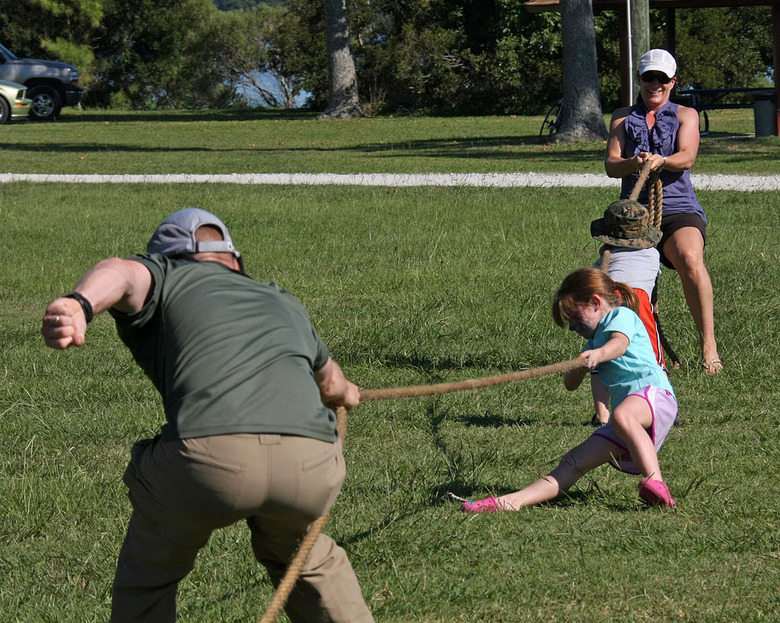# Self Check Review# Critique

Review your work on the Self Check problem and think about the following questions.

• What can you do to both sides of the equation to get x by itself on one side?
• What property of equality can you use to solve the equation?
• Substitute your solution back into the original equation. Do you get a true equation?
• Can you think of a real-world situation about the height of something, the cost of something, or earning money?
• Can you think of a proportional situation for the last equation?
1 of 7